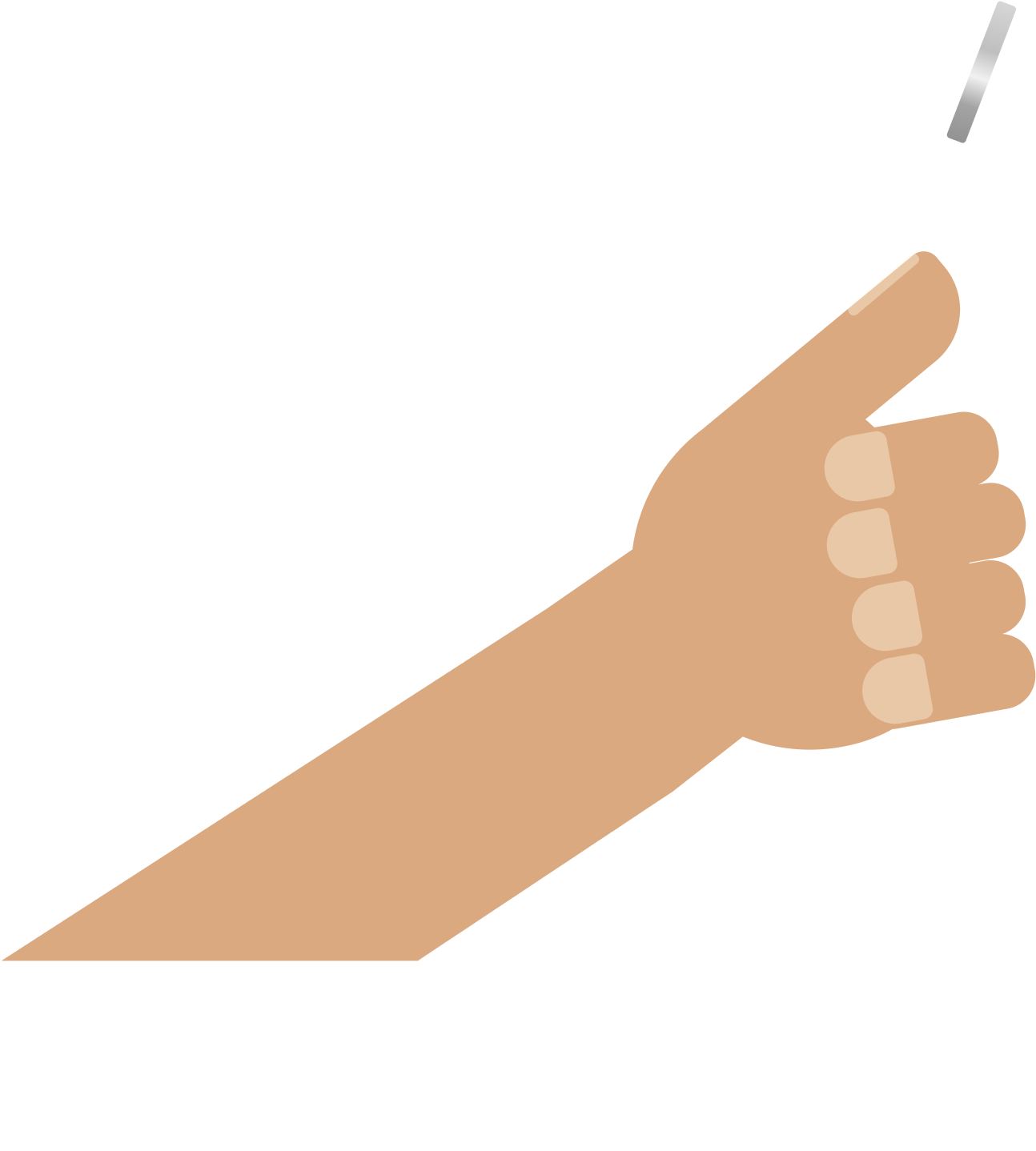# An Extremely Biased Coin - I

Probability Level 2

You have an (extremely) biased coin that shows heads with probability 99% and tails with probability 1%. To test the coin, you tossed it 100 times.

What is the approximate probability that heads showed up exactly $99$ times?×